### Magnetic Effects of Electric Current - Revision Notes

CBSE Class 10 Science

Revision Notes
CHAPTER – 13
MAGNETIC EFFECTS OF ELECTRIC CURRENT

In this chapter, we will study the effects of electric current : Moving charges or electric current generates a magnetic field.

1. Hans Christian Oersted (1777-1851)
Oersted showed that electricity and magnetism are related to each other.His research later used in radio, television etc.
The unit of magnetic field strength is named Oersted in his honour.

2. Oersted Experiment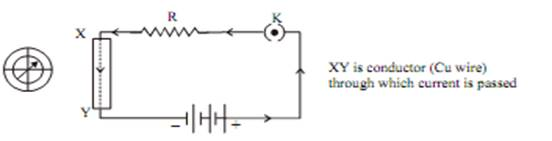On passing the current through the copper wire XY in the circuit, the compass needle which is placed near the conductor gets deflected. If we reverse the direction of current, the compass needle deflect in reverse direction. If we stop the flow of current, the needle comes at rest.
Hence, it can be concluded that electricity and magnetism are linked to each other. It shows that whenever the current will flow through the conductor,then a magnetic field will develop.

3. Magnetic Field : It is the region surrounding a magnet, in which force of magnet can be detected. It is a vector quantity, having both direction& magnitude.

4. Compass Needle : It is a small bar magnet, whose north end is pointing towards north pole and south end is pointing towards south pole of earth.

5. Magnetic Field Lines : The tangent to the magnetic field line at a point gives the direction of magnetic field at that point.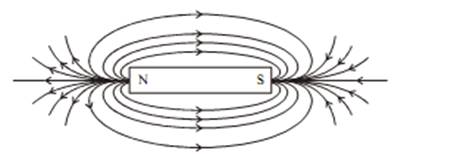Hence, magnetic field line is a path along which a hypothetical free north pole tend to move towards south pole.

6. Characteristics of Magnetic field lines :

(a) The direction of magnetic field lines outside the magnet is always from north pole to south pole of bar magnet and are indicated by an arrow.Inside the magnet, the direction of field lines is from its south pole to north pole. Thus magnetic field lines are closed curves.
(b) The strength of magnetic field is expressed by the closeness of magnetic field lines. Closer the lines, more will be the strength and farther the lines, less will be the magnetic field strength.
(c) No two field lines will intersect each other.If they intersects, then at point of intersection the compass needle will show two directions of magnetic field which is not possible.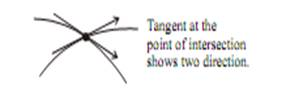7. Magnetic field due to Current Carrying Conductor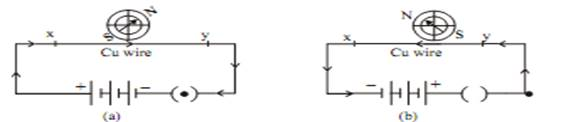The above electric circuit in which a copper wire is placed parallel to a compass needle, shows the deflection in needle gets reversed, when the direction of current reversed. Hence electricity and magnetism are related to each other.

8. Right Hand Thumb Rule

It is a convenient way of finding the direction of magnetic field associated with current carrying conductor. Hold the straight wire carrying current in your right hand such that thumb points towards the direction of current, then your folded fingers around the conductor will show the direction of magnetic field.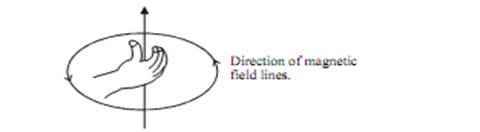This rule also called Maxwell’s corkscrew rule.

9. Magnetic Field due to Current through a Straight Conductor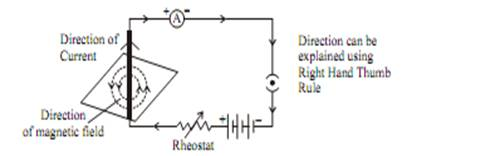Let a current carrying conductor be suspended vertically and the electric current is flowing from south to north. In this case, the direction of magnetic field will be anticlockwise. If the current is flowing from north to south, the direction of magnetic field will be clockwise.

A current carrying straight conductor has magnetic field in the form of concentric circles; around it. Magnetic field of current carrying straight conductor can be shown by magnetic field lines.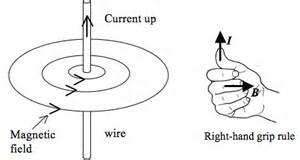10. Magnetic Field due to Current through a circular Loop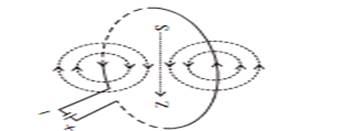Every point on the wire carrying current give rise to the magnetic field,appearing as a straight line at the centre of loop. By applying Right hand Thumb rule, we can find the direction of magnetic field at every section of the wire.

11. Solenoid :A Coil of many circular turns of insulated copper wire wrapped closely in the shape of a cylinder is called solenoid.

12. Magnetic field due to a current in a solenoid :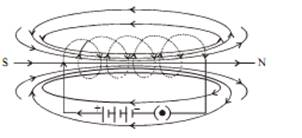• Using R.H. Thumb Rule, we can draw the pattern of magnetic field lines around a current carrying ‘Solenoid’.
• One end of the solenoid behaves as a magnetic north pole,while the other end behave as the South Pole.
• The filed lines inside the solenoid are in form of parallel straight lines, that implies that magnetic field inside the solenoid is same at all points i.e. Field is uniform.

The strength of the magnetic field produced depends upon
(a) the number of turns
(b) Strength of current in the solenoid used in making solenoid.

13. Electromagnet : Strong magnetic field inside the solenoid can be used to magnetise a magnetic material for example soft iron, when it is placed inside the coil. The magnet so formed is called electromagnet.It is a temporary magnet.
Properties of Magnetic Field:

• The magnitude; of magnetic field increases with increase in electric current and decreases with decrease in electric current.
• The magnitude of magnetic field; produced by electric current; decreases with increase in distance and vice-versa. The size of concentric circles of magnetic field lines increases with distance from the conductor, which shows that magnetic field decreases with distance.
• Magnetic field lines are always parallel to each other.
• No two field lines cross each other.

14. Force on a current carrying conductor in a magnetic field.
Andre Marie Ampere (1775–1836) suggested that the magnet also exert an equal and opposite force on the current carrying conductor.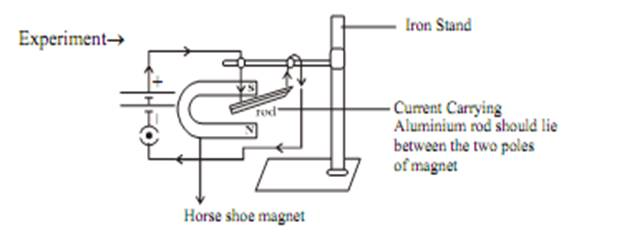We will observe that the rod will displace i.e. the rod will experience a force, when it is placed in magnetic field, in a perpendicular direction to its length.

• The direction of the exerted force will be reversed if the direction of current through the conductor is reversed.
• If we change the direction of field by inter changing the two poles of the magnet, again the direction of exert force will change.
• Therefore the direction of exerted force depends on

(a) direction of current
(b) direction of magnetic field lines.

15. Left Hand fleming Rule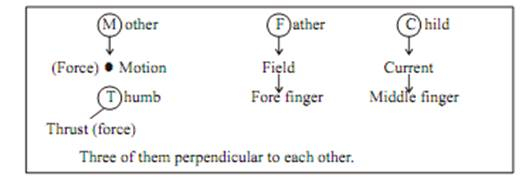OR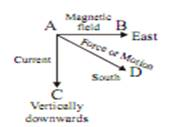• According to this rule, stretch thumbforefinger, and middle finge rof your left hand such that they are mutually perpendicular to each other.

If fore finger represent direction of magnetic field & middle finger represent direction of current,then thumb will point in the direction of motion or force acting on the conductor.

– ELECTRIC MOTOR :

Electrical energy is converted into mechanical energy by using an electric motor. Electric motor works on the basis of rule suggested by Marie Ampere and Fleming’s Left Hand Rule.

In an electric motor, a rectangular coil is suspended between the two poles of a magnetic field. The electric supply to the coil is connected with a commutator. Commutator is a device which reverses the direction of flow of electric current through a circuit.

When electric current is supplied to the coil of electric motor, it gets deflected because of magnetic field. As it reaches the half way, the split ring which acts as commutator reverses the direction of flow of electric current. Reversal of direction of current reverses the direction of forces acting on the coil. The change in direction of force pushes the coil; and it moves another half turn. Thus, the coil completes one rotation around the axle. Continuation of this process keeps the motor in rotation.

In commercial motor, electromagnet; instead of permanent magnet; and armature is used. Armature is a soft iron core with large number of conducting wire turns over it. Large number of turns of conducting wire enhances the magnetic field produced by armature.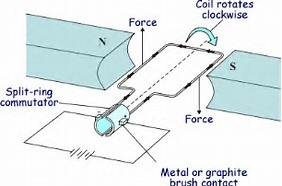16. Michael Faraday : Gave the law of Electro magnetic Induction : When a conductor is set to move inside a magnetic field or a magnetic field is set to be changing around a conductor, electric current is induced in the conductor.

17. Galvanometer : It is an instrument that can detect the presence of a current in a circuit. If pointer is at zero (the centre of scale) then there will be no flow of current.
If the pointer deflect on either side right or left, this will show the direction of current. Represented by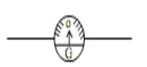ELECTRIC GENERATOR :

The structure of electric generator is similar to that of an electric motor. In case of an electric generator a rectangular armature is placed within the magnetic field of a permanent magnet. The armature is attached to wire and is positioned in way that it can move around an axle. When the armature moves within the magnetic field an electric current is induced. The direction of induced current changes, when the armature crosses the halfway mark of its rotation. Thus, the direction of current changes once in every rotation. Due to this, the electric generator usually produces alternate current, i.e. AC. To convert an AC generator into a DC generator, a split ring commutator is used. This helps in producing direct current.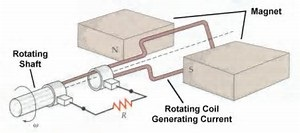18. Electro Magnetic Induction: Can be explained by two experiments

(a) First Experiment $\to$ “Self Induction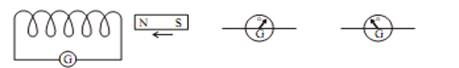In this experiment, when the north pole of bar magnet is brought closer to the coil or away from the coil, we see momentary deflection in the needle of galvanometer on either side of null point. First right and then left.

Similarly, if we keep the magnet stationary and coil is made to move towards or away from the north pole of magnet. Again we will observe deflection in the needle of galvanometer.

If both bar magnet and coil are kept stationary, there will be no deflection in galvanometer. This experiment can also be done with the south pole of magnet, we will observe the deflection in galvanometer, but it would be in opposite direction to the previous case.

$⇒$ It concludes that motion of magnet with respect to coil or vice-versa, changes the magnetic field. Due to this change in magnetic field lines, potential difference is induced in the same coil, which set up an induced current in the circuit.

(b) Second Experiment : Mutual Induction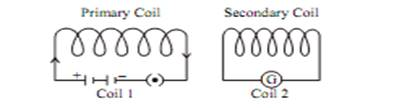In this experiment plug in the key that connects coil with battery and observe the deflection in galvanometer. Now plug out the key that disconnect the coil-1 from battery and observe the deflection in galvanometer, which will be in reverse direction.

Hence, we conclude that potential difference is induced in secondary coil (coil-2), whenever there is a change in current, in primary coil(coil-1) (by on and off of key).

This is because, whenever there is change in current in primary coil

$\phantom{\rule{thickmathspace}{0ex}}\phantom{\rule{thickmathspace}{0ex}}\phantom{\rule{thickmathspace}{0ex}}\phantom{\rule{thickmathspace}{0ex}}\phantom{\rule{thickmathspace}{0ex}}↓$

Magnetic field associated with it also changes

$\phantom{\rule{thickmathspace}{0ex}}\phantom{\rule{thickmathspace}{0ex}}\phantom{\rule{thickmathspace}{0ex}}\phantom{\rule{thickmathspace}{0ex}}\phantom{\rule{thickmathspace}{0ex}}↓$

Now, magnetic field lines around the secondary coil (coil-2) will change and induces the electric current in it (observed by the deflection of needle of Galvanometer in secondary circuit)

This process, by which changing of strength of current in primary coil, induces a current in secondary coil is called Electromagnetic Induction”

The induced current is found to be highest when the direction of motion of coil is at right angles to the magnetic field.

19. Fleming’s Right Hand Rule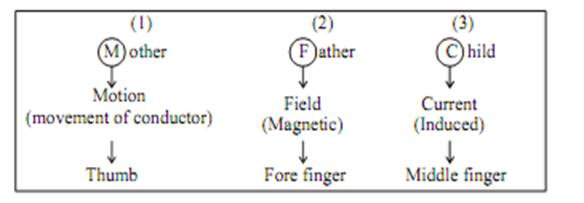OR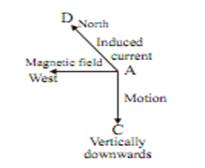Rule can be defined as :

Stretch, thumb, forefinger, and middle finger of right hand, so that they are perpendicular to each other. The forefinger indicates direction of magnetic field, thumb shows the direction of motion of conductor, then the middle finger will shows the direction of induced current.

Electrical generator is based on the principle of electromagnetic induction. It convert mechanical energy into electrical energy.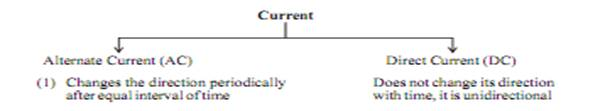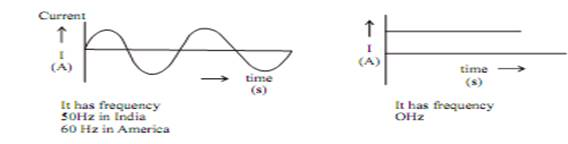21. Advantages of Alternate Current (AC) over Direct Current (DC)

Electric power can be transmitted to longer distances without much loss of energy. Therefore cost of transmission is low.

In India the frequency of AC is 50Hz. It means after every 1/100 second it changes its direction.

22. Domestic Electric Circuits :

In our homes, the electric power supplied is of potential difference V = 220V and frequency 50Hz.

It consist of three wires :–
(1) Wire with red insulation cover – LIVE WIRE (POSITIVE) Live wire is at high potential of 220V
(2) Wire with black insulation cover – NEUTRAL WIRE(NEGATIVE) Neutral wire is at zero potential Therefore, the potential difference between the two is 220V.
(3) Wire with Green insulation cover – EARTH WIRE
It is connected to a copper plate deep in the earth near house.
The metallic body of the appliances is connected with the earth wire as a safety measure.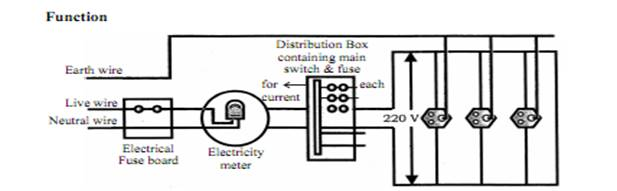Earth wire provide a low resistance to the current hence any leakage of current to the metallic body of the appliances, keep its potential equal to that of earth. That means zero potential and the user is saved from severe electric shock.

Point to be noted in Domestic Circuit
(a) Each appliance has a separate switch of ON/OFF
(b) In order to provide equal potential difference to each appliance, they should be connected parallel to each other. So that they can be operated at any time.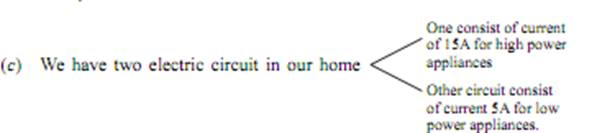24. Short Circuiting : Due to fault in the appliances or damage in the insulation of two wires, the circuit will offer zero or negligible resistance to the flow of current. Due to low resistance, large amount of current will flow.
According to Joule’s law of heating effect , heat is produced in live wire and produces spark, damaging the device and wiring.

25. Overloading : Overloading can be caused by (1) Connecting too many appliances to a single socket or (2) accidental rise in supply voltage if the total current drawn by the appliances at a particular time exceeds the bearing capacity of that wire, it will get heated up. This is known as overloading. Fuse a safety device can prevent the circuit from overloading and short circuiting.

What you have learnt

• A compass needle is a small magnet. Its one end, which points towards north, is called a north pole, and the other end, which points towards south, is called a south pole.
• A magnetic field exists in the region surrounding a magnet, in which the force of the magnet can be detected.
• Field lines are used to represent a magnetic field. A field line is the path along which a hypothetical free north pole would tend to move. The direction of the magnetic field at a point is given by the direction that a north pole placed at that point would take. Field lines are shown closer together where the magnetic field is greater.
• A metallic wire carrying an electric current has associated with it a magnetic field.The field lines about the wire consist of a series of concentric circles whose direction is given by the right-hand rule.
• The pattern of the magnetic field around a conductor due to an electric current flowing through it depends on the shape of the conductor. The magnetic field of a solenoid carrying a current is similar to that of a bar magnet.
• An electromagnet consists of a core of soft iron wrapped around with a coil of insulated copper wire.
• A current-carrying conductor when placed in a magnetic field experiences a force.If the direction of the field and that of the current are mutually perpendicular to each other, then the force acting on the conductor will be perpendicular to both and will be given by Fleming’s left-hand rule. This is the basis of an electric motor.An electric motor is a device that converts electric energy into mechanical energy.
• The phenomenon of electromagnetic induction is the production of induced current in a coil placed in a region where the magnetic field changes with time. The magnetic field may change due to a relative motion between the coil and a magnet placed near to the coil. If the coil is placed near to a current-carrying conductor, the magnetic field may change either due to a change in the current through the conductor or due to the relative motion between the coil and conductor. The direction of the induced current is given by the Fleming’s right-hand rule.
• A generator converts mechanical energy into electrical energy. It works on the basis of electromagnetic induction.
• In our houses we receive AC electric power of 220 V with a frequency of 50 Hz. One of the wires in this supply is with red insulation, called live wire. The other one is of black insulation, which is a neutral wire. The potential difference between the two is 220 V.

The third is the earth wire that has green insulation and this is connected to a metallic body deep inside earth. It is used as a safety measure to ensure that any leakage of current to a metallic body does not give any severe shock to a user.

• Fuse is the most important safety device, used for protecting the circuits due to short-circuiting or overloading of the circuits.